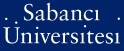# Fuzzy linear programming: review and implementation

Dervişoğlu Toprak, Esra (2005) Fuzzy linear programming: review and implementation. [Thesis]PDF - Registered users only - Requires a PDF viewer such as GSview, Xpdf or Adobe Acrobat Reader4002Kb

## Abstract

Most of the time we encounter problems where quantities cannot be expressed by crisp numbers; instead, the use of vague qualifications is preferred. The Fuzzy Set Theory (FST), developed by Lotfi A. Zadeh, is an effective framework which can be used in the solution of such problems. In FST, the objects belong to a set to a degree between [0,1]. The degree of belongingness is referred to as the membership degree. FTS states that utilizing this membership degree is more profitable than just partitioning the objects dichotomously as in classical bivalent (two-valued) set theory. In most of the problems, where linear programming can be applied , the decision maker choses to state the inequalities and the coefficients used in objective function and constraints by vague expressions. Fuzzy Linear Programming, based on the FST, is developed to model and solve such problems. In this thesis, the proposed aproach, in the most general case, first compares the fuzzy left-hand side with the fuzzy right-hand side and the fuzzy objective function with a fuzzy goal by means of a membership function based on the fuzzy relation using "min" function. After determination of these membership functions associated with the constraints and the objective function, a new auxiliary problem is formed. The obtained auxiliary problem is a non-linear fractional programming problem with its nominator are defined by linear functions. The optimal solution of such a problem can be found by solving a sequence of linear programs. In this study, the solution approaches present in the literature for fuzzy linear programming are categorized, some points which are unclear is identified and tried to be improved, and finally the proposed solution methodology is applied to so-called fuzzy analytical hierarchy process.

Item Type: Thesis Fuzzy linear programming -- Analytical hierarchy process Q Science > QA Mathematics 8263 IC-Cataloging 15 Apr 2008 10:47 27 Dec 2008 12:20

Repository Staff Only: item control page